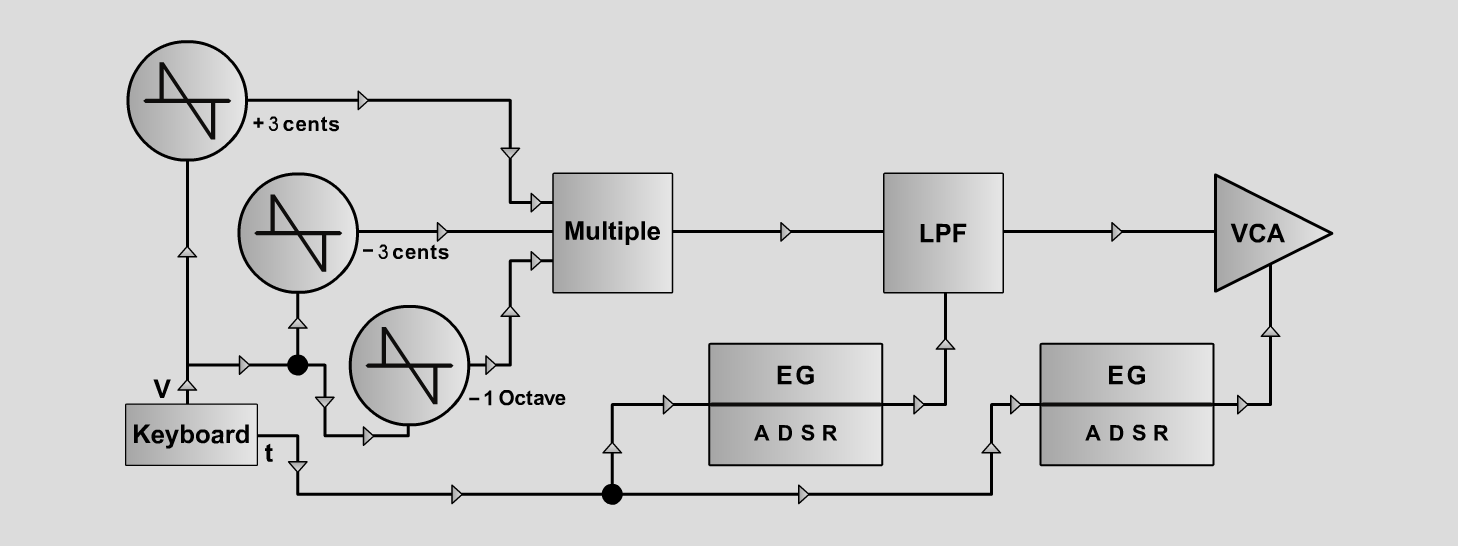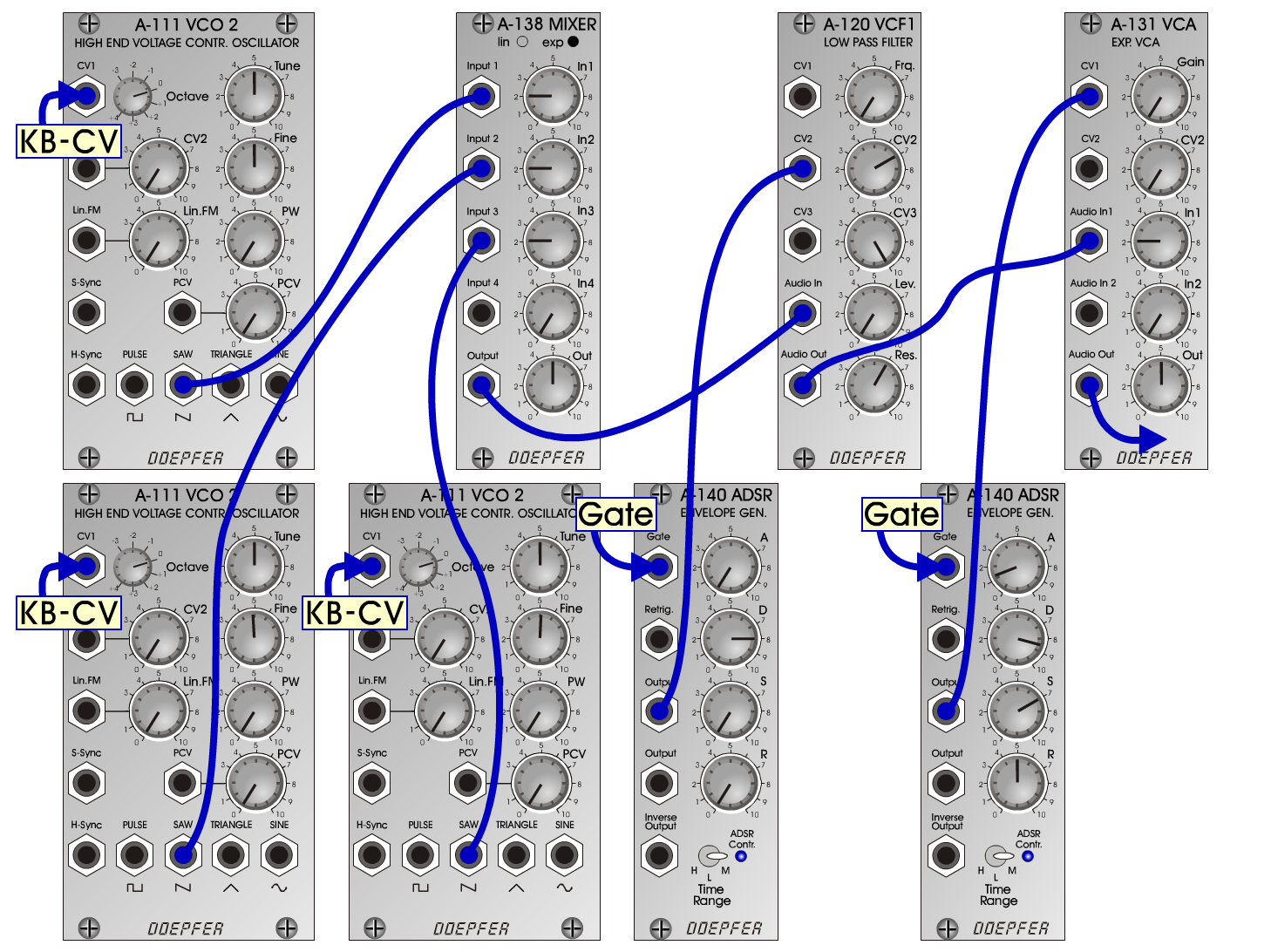Moogli-BassInstrumentsD o e p f e r A - 100 Connections: Settings: Moogli-Bass1 A-111/1 (Saw) <=> A-138exp (Input 1)  A-111/2 (Saw) <=> A-138exp (Input 2)  A-111/3 (Saw) <=> A-138exp (Input 3)  A-138exp (Output) <=> A-120 (Audio In)  A-140/1 (Output) <=> A-131 (CV1)  A-140/2 (Output) <=> A-120 (CV2)  A-120 (Audio Out) <=> A-131 (Audio In)  A-131 (Audio Out) <=> Amp A-138p (In 1 = 2, In 2 = 2, In 3 = 2, Out = 5)  A-120 (Freq = 0, CV2 = 7, Lev = 10, Res = 6)  A-140/1 (A = 1.3, D = 8.5, S = 7, R = 5, Range = M)  A-140/2 (A = 0, D = 8, S = 0, R = 0, Range = M)  A-131 (Gain = 0, Audio In 1 = 5, Audio Out = 7) Moogli-Bass2 A-138p (In 1 = 2, In 2 = 2, In 3 = 2, Out = 5)  A-120 (Freq = 0, CV2 = 8, Lev = 10, Res = 6)  A-140/1 (A = 1.0, D = 8.5, S = 7, R = 5, Range = M)  A-140/2 (A = 0, D = 8, S = 0, R = 0, Range = M)  A-131 (Gain = 0, Audio In 1 = 5, Audio Out = 7) Moogli-Bass3 A-138p (In 1 = 2, In 2 = 2, In 3 = 2, Out = 5)  A-120 (Freq = 2, CV2 = 6, Lev = 8, Res = 6.5)  A-140/1 (A = 1.3, D = 8.5, S = 7, R = 5, Range = M)  A-140/2 (A = 0, D = 7, S = 0, R = 0, Range = M)  A-131 (Gain = 0, Audio In 1 = 2, Audio Out = 5) Notes: Tuning: A-111/1 = -1 octave, A-111/2 = -3 cents, A-111/3 = +3 cents Josef MuellerSound samples Bach Passacaglia (BWV 582)      Second instrument: E-Organ Moogli-Bass 1 A Moogli-Bass 1 B Moogli-Bass 1 C Moogli-Bass 2 A Moogli-Bass 2 B Moogli-Bass 2 C Moogli-Bass 3 A Moogli-Bass 3 B Moogli-Bass 3 C